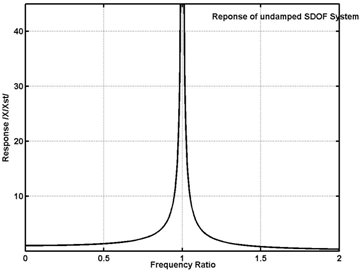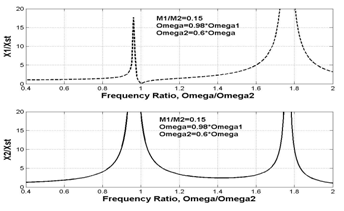# Tuned Vibration Absorber

## Aim

Tuned Vibration Absorber

Many–a-times, a vibratory system under forced vibration is required to run near resonance i.e. the excitation frequency is close to the natural frequency of the system. Under such a situation, the response of the system can be large and we must try to reduce it by taking some measure. The excitation frequency may be constant in many cases as in case of a machine with rotor-unbalance running at constant speed. By attaching a separate smaller spring-mass system, an auxiliary system, to the main system the vibration of the main system can be reduced, drastically, if the mass and the stiffness of the auxiliary system are properly calculated, i.e. if the auxiliarysystem is tuned to the natural frequency of the main system and the excitation frequency. Underthis condition,thevibrationof the main system is reduced almost to zero and the auxiliary system vibrates in response to the excitation. So, it is termed as vibration absorber and this method of vibration control is called vibration absorption.

In this experiment, you will try to design a tuned vibration absorber. You will also study the effect of changing the mass and stiffness of an auxiliary system (i.e. natural frequency of an auxiliary system when it is isolated) on response of the main system to a harmonic force. The harmonic force is considered to be generated out of unbalanced rotating mass with constant rpm. The mass and stiffness of main system are considered to be fixed which is usually the case in practical situation also. The frequency of theexcitation force and natural frequency of main system are close to each other. Thus, we have a main system with fixed natural frequency and a harmonic excitation force having frequency closer to the natural frequency of the main system. In other words, we have a system running at or near resonance having a large response, naturally, which will be reduced by attaching a properly tuned vibration absorber. Damping is neglected for simplicity and ease of understanding.

Follow the procedure given on another screen which is opened by clicking the tab ‘Procedure’ and use the simulator later to perform the experiment.

Theory

The theory on which thedesign of tuned vibration absorber is based is as given below:

The response of a SDOF system, subjected to a harmonic force having frequency close to the natural frequency of the system, will be quite large when the frequencies are equal. It will be infinite, theoretically, if damping is neglected. The variation of amplitude of response with respect to the excitation frequency is as shown in the graph below.Undamped SDOF system with harmonic force excitationResponse of undamped SDOF system to harmonic force; Magnitude of response v/s frequency of excitation, ω/ωn

For an undamped SDOF system , the forced response is given bywhere, Xst is the static deflection of the spring which is equal to the ratio of amplitude of the excitation force, F0,and the stiffness of the spring, K.
ω - Excitation frequency,
ωn- Naturalfrequency of the system.
At ω = 0.95ωn, we get X/Xst = 10.26. The plot of the amplitude of response, X/Xst , versus frequency ratio, ω/ωn, is shown in the figure above.
Now, we attach an auxiliary SDOF system with mass M2 and stiffness K2 to the main system which has a mass M1 and stiffness K1. When these systems were independent (separate), they would have their own natural frequencies given by ωn1 = (K1/M1)^0.5 for the main system, and ωn2 = (K1/M2)^0.5 for the auxiliary system
It is to be noted that on attaching the auxiliary system to main system, another system is formed which is now a two degree of freedom system. It will have its own two natural frequencies but quite different from ω1 and ω2 that we considered above.
The response of each mass of the system, now a 2-DOF system, to harmonic force acting on M1 (Amplitude of vibration of each mass is given by), is given byμ is the mass ratio = M2/M1. ωn1 = (K1/M1)^0.5 and ωn2 = (K1/M2)^0.5, However, the natural frequencies of the 2-degree of freedom system formed after attaching the auxiliary system are given by different expressions in terms of M1, M2, K1, and K2. Plot of the dimensionless responses of each of the two masses is shown above when a harmonic force acts on M­1 when excitation frequency is close to natural frequency of main system,ω ≠ω1 ,, but much away from natural frequency of auxiliary system,ω ≠ω2, Mass ratio μ=M2/M1= 0.15.]-->Main system attached with an auxiliary system – Now, a 2-DOF system Frequency response of each of the two masses when a harmonic force acts on M­1 when excitation frequency is close to natural frequency of main system ω ]-->≈ ω1 but much away from natural frequency of auxiliary system, ω ≈ ω2. Mass ratio μ=M2/M1= 0.15.

Now we consider that excitation frequency is almost equal to the natural frequency of the main system alone. Because of this, its response would be large before attaching the auxiliary system. If we attach the auxiliary system such that its natural frequency is equal to the excitation frequency, and hence also equal to that of the main system, and substitute ω =ω12 in above equations, we find that X1 reduces to zero and X2 = F0/(μ.K1), XST = F0/K1. Thus, by attaching an auxiliary system with natural frequency equal to the excitation frequency and natural frequency of the main system makes the response of the main system zero though the auxiliary system oscillates with anamplitude equal to F0/(μ.K1).And this is the principle of working of a vibration absorber.
While designing the vibration absorber, we must select K2 and M2 such that ω=ω2 = (K2/M2)^0.5 and to keep response of auxiliary system minimum, we must have X2 = F0/(μ.K1) minimum. As F0 and K1 cannot be changed, adjusting the mass ratio =M2/M1(in fact, only M2) is in our hand. For smaller X2, we should have large M2 and we cannot increase M2 to a very large extent because the auxiliary system should not be bulky. So, a compromise is made and value of μ is kept in the range of 0.05 to 0.25. Observe this in the figure belowIn this experiment, while the auxiliary system is tuned, you would choose different values of mass ratio and observe the responses of the primary and secondary systems. The responses can also be observed even if the auxiliary system is not tuned i.e. when the natural frequency of auxiliary system is not equal to the excitation frequency or the natural frequency of the main system. This is done by choosing values of M2 and K2 such that ω ≠ ω2 as ω2 = (K2/M2)^0.5.